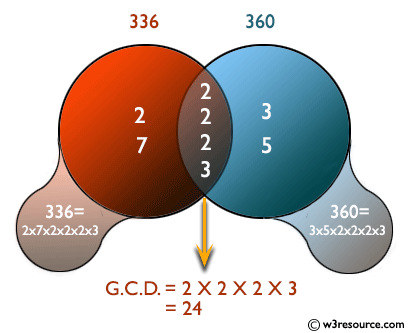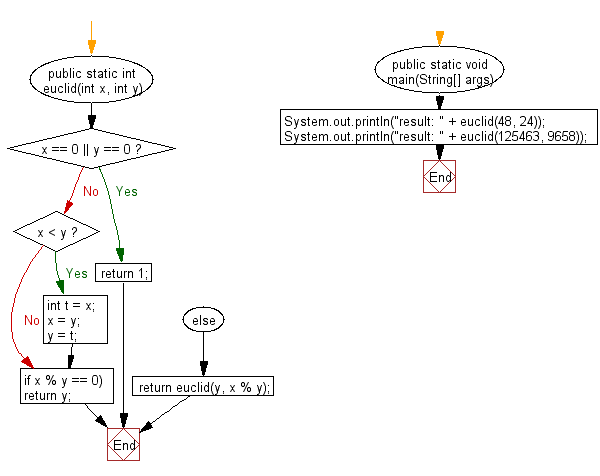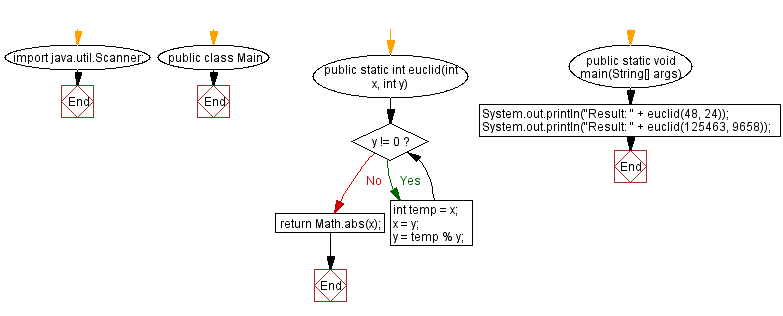﻿ Java - Euclid algorithm, find the GCD of two integers

# Java: Prove that Euclid’s algorithm computes the greatest common divisor of two positive given integers

## Java Basic: Exercise-157 with Solution

Write a Java program to prove that Euclid’s algorithm computes the greatest common divisor of two integers that have positive values.

According to Wikipedia "The Euclidean algorithm is based on the principle that the greatest common divisor of two numbers does not change if the larger number is replaced by its difference with the smaller number. For example, 21 is the GCD of 252 and 105 (as 252 = 21 × 12 and 105 = 21 × 5), and the same number 21 is also the GCD of 105 and 252 − 105 = 147. Since this replacement reduces the larger of the two numbers, repeating this process gives successively smaller pairs of numbers until the two numbers become equal. When that occurs, they are the GCD of the original two numbers. By reversing the steps, the GCD can be expressed as a sum of the two original numbers each multiplied by a positive or negative integer, e.g., 21 = 5 × 105 + (−2) × 252. The fact that the GCD can always be expressed in this way is known as Bézout's identity."

Pictorial Presentation:Sample Solution-1:

Java Code:

``````import java.util.Scanner;
public class Solution {
public static int euclid(int x, int y) {
if (x == 0 || y == 0) {
return 1;
}
if (x < y) {
int t = x;
x = y;
y = t;
}
if (x % y == 0) {
return y;
} else {
return euclid(y, x % y);
}
}

public static void main(String[] args) {
System.out.println("result: " + euclid(48, 24));
System.out.println("result: " + euclid(125463, 9658));
}
}
```
```

Sample Output:

```result: 24
result: 1
```

Flowchart:Sample Solution-2:

Java Code:

``````import java.util.Scanner;
public class Main {
public static int euclid(int x, int y){
while (y != 0){
int temp = x;
x = y;
y = temp % y;
}
return Math.abs(x);
}       public static void main(String[] args) {
System.out.println("Result: " + euclid(48, 24));
System.out.println("Result: " + euclid(125463, 9658));
}
}
```
```

Sample Output:

```Result: 24
Result: 1
```

Flowchart:Java Code Editor: